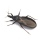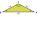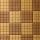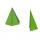# Planimetrics - math word problems

Study plane measurements, including angles, distances, and areas. In other words - measurement and calculation of shapes in the plane. Perimeter and area of plane shapes.

#### Number of problems found: 2136

• Four sides of trapezoidCalculate the area of the ABCD trapezoid with sides a = 65 cm, b = 29 cm, c = 40 cm, d = 36 cm
• Company logoThe company logo consists of a blue circle with a radius of 4 cm, which is an inscribed white square. What is the area of the blue part of the logo?
• Angles of the triangleABC is a triangle. The size of the angles alpha, beta are in a ratio 4: 7. The angle gamma is greater than the angle alpha by a quarter of a straight angle. Determine angles of the triangle ABC.
• SquaresCalculate the area of square and result round to square decimeters. a) a = 1,52 dm b) a = 13 268mm c) a = 562 cm d) a = 1,52 m
• The ladderThe ladder touch on a wall at a height of 7.5 m. The angle of the inclination of the ladder is 76°. How far is the lower end of the ladder from the wall?
• A bugA bug was sitting on the tip of a wind turbine blade that was 24 inches long when it started to rotate. The bug held on for 5 rotations before flying away. How far did the bug travel before it flew off? Exact answer.
• Two chordsFrom the point on the circle with a diameter of 8 cm, two identical chords are led, which form an angle of 60°. Calculate the length of these chords.
• Roof angleThe roof of the house has the shape of an isosceles triangle with arms 4 m long and the size of the base 6 m. How big an angle alpha does its roof make?
• Cube diagonalsDetermine the volume and surface area of the cube if you know the length of the body diagonal u = 216 cm.
• TilesHow many square tiles with an area of 121 cm2 have to be ordered for the square room's paving with a side length of 2.75 meters?
• TentPyramid-shaped tent has a base square with a side length of 2 m and a height 1.7 m. How many meters of canvas is nneded to make it if for a waste should be added 10%?
• Climb in percentageThe height difference between points A and B is 475 m. Calculate the percentage of route climbing if the horizontal distance places A, B is 7.4 km.
• TilesHall has dimensions 325 &time; 170 dm. What is the largest size of square tiles that can be entire hall tiled and how many we need them?
• RT and ratioA right triangle whose legs are in a ratio 6:12 has hypotenuse 68 m long. How long are its legs?
• ClocksHow will long path walk the end of the clock's minute-hand long 7 cm for 330 minutes? How long will walk in the same time hour-hand?
• Cylinder - hThe cylinder volume is 140 cm3. The base radius is 7 cm. Calculate the height of the cylinder.
• Maximum of volumeThe shell of the cone is formed by winding a circular section with a radius of 1. For what central angle of a given circular section will the volume of the resulting cone be maximum?
• Sphere slicesCalculate the volume and surface of a sphere if the radii of parallel cut r1=31 cm, r2=92 cm, and its distance v=25 cm.
• Map scaleThe rectangular plot has a scale of 1: 10000 area 3 cm2 on the map. What content does this plot have on a 1:5000 scale map?
• SectorThe perimeter of a circular sector with an angle 1.8 rad is 64 cm. Determine the radius of the circle from which the sector comes.

Do you have an interesting mathematical word problem that you can't solve it? Submit a math problem, and we can try to solve it.

We will send a solution to your e-mail address. Solved examples are also published here. Please enter the e-mail correctly and check whether you don't have a full mailbox.

Please do not submit problems from current active competitions such as Mathematical Olympiad, correspondence seminars etc...

See also more information on Wikipedia.